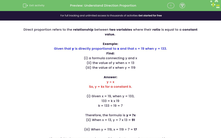# Understand Direction Proportion

In this worksheet, students will be asked to resolve problems related to direct proportion.Key stage:  KS 3

Curriculum topic:   Ratio, Proportion and Rates of Change

Curriculum subtopic:   Solve Problems Involving Direct and Inverse Proportion

Difficulty level:#### Worksheet Overview

Direct proportion refers to the relationship between two variables where their ratio is equal to a constant value.

Example:

Given that y is directly proportional to x and that x = 19 when y = 133.

Find:
(i) a formula connecting y and x
(ii) the value of y when x = 13
(iii) the value of x when y = 119

y ∝ x

So, y = kx for a constant k.

(i) Given x = 19, when y = 133,

133 = k x 19

k = 133 ÷ 19 = 7

Therefore, the formula is y = 7x

(ii) When x = 13, y = 7 x 13 = 91

(iii) When y = 119, x = 119 ÷ 7 = 17

It's useful to have paper and a pencil in order to record your own workings.

These are not the kind of questions you can calculate mentally.

Over to you!

### What is EdPlace?

We're your National Curriculum aligned online education content provider helping each child succeed in English, maths and science from year 1 to GCSE. With an EdPlace account you’ll be able to track and measure progress, helping each child achieve their best. We build confidence and attainment by personalising each child’s learning at a level that suits them.

Get started